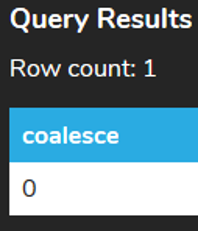+

# SUM to Add Values

##### Rating:
(0)
Author: Sophia Tutorial
##### Description:

Given a table with currency based values (such as employee salary) use the SUM function to compose a query that provides the total of a given subset of records within the table.

(more)
Tutorial

what's covered
This tutorial explores using the SUM function to add values within a column together in two parts:
1. Getting Started
2. Special Cases

## 1. Getting Started

SUM is an aggregate function that returns the sum of all of the values in a column given a filter. Similar to the COUNT function, the SUM function ignores NULL values in a column. The SUM function is used on numeric columns. For example, if we would like to find the sum of the total amount for all the invoices, then we run the following:

``````
SELECT SUM(total)
FROM invoice;
``````

This would SUM the values in the total column that are not NULL, which would give the result:We may also want to SUM based on certain criteria to be restricted by using the WHERE clause. For example, we could find the SUM of the total amount for all invoices in the USA:

``````
SELECT SUM(total)
FROM invoice
WHERE billing_country = 'USA';
``````Anything that we can filter with the WHERE clause, we can then aggregate to find the SUM for.

Similar to the COUNT function, we can also use the DISTINCT option where it will only calculate the SUM of the DISTINCT values. For example, if we had a total of 1, 2, 2, and 4, and we used the regular SUM function, the function would return 9. However, if we use DISTINCT, the query will return 7, as 2 was repeated.

## 2. Special Cases

If we tried to use the SUM on a non-numeric column, this would result in an error:

``````
SELECT SUM(billing_country)
FROM invoice;
``````If the query returns only NULL values or if no rows are returned, the SUM returns NULL rather than 0. For example, if we’re looking for the SUM of all of the invoices that have the invoice_id < 1 (which does not exist):

``````
SELECT SUM(total)
FROM invoice
WHERE invoice_id < 1;
``````

The result will look like this:If we wanted to return a 0 instead of NULL in this case, we would have to use the COALESCE function. This function returns the second argument if the first argument is NULL. For example:

``````
SELECT COALESCE(SUM(total),0)
FROM invoice
WHERE invoice_id < 1;
``````try it
Your turn! Open the SQL tool by clicking on the LAUNCH DATABASE button below. Then enter in one of the examples above and see how it works. Next, try your own choices for which columns you want the query to provide.

summary
The SUM function returns the sum of the values in a column without NULL values.

Source: Authored by Vincent Tran

Rating Header